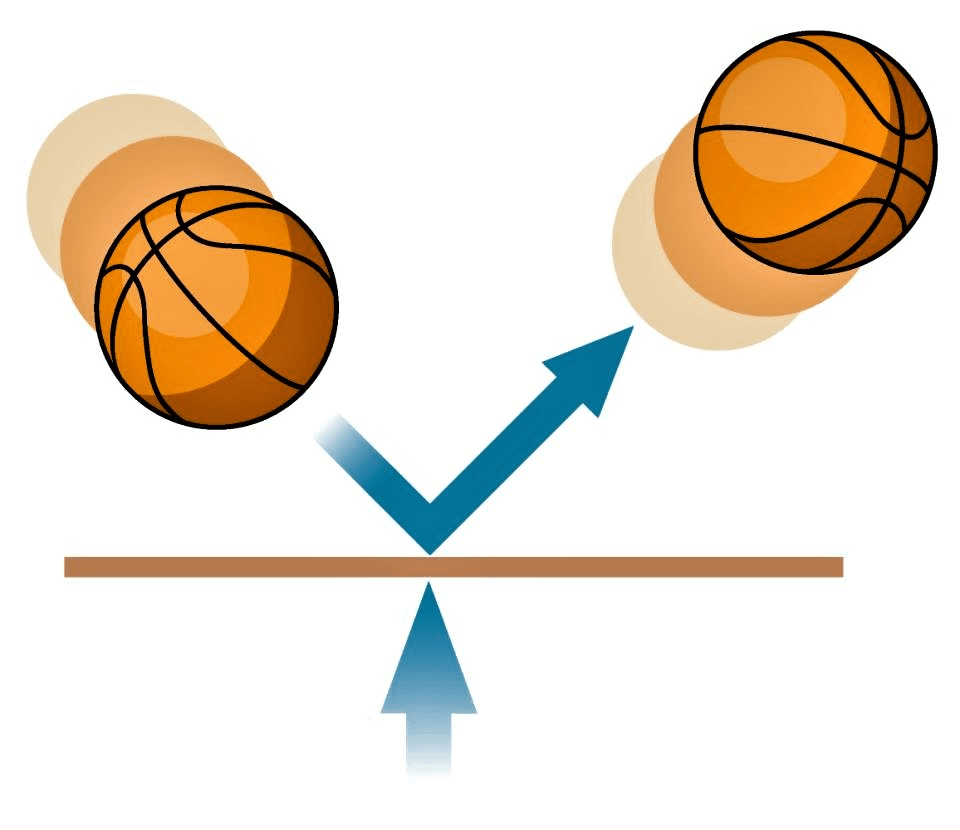Courses

# Important Notes - Force & Pressure, Science, Class 8 | EduRev Notes

## Class 8 : Important Notes - Force & Pressure, Science, Class 8 | EduRev Notes

The document Important Notes - Force & Pressure, Science, Class 8 | EduRev Notes is a part of the Class 8 Course Science Class 8.
All you need of Class 8 at this link: Class 8

 1.    A push or a pull acting on an object is called a force 2.    Force has magnitude as well as direction. 3.    A force between the two objects only when they interact with each other. 4.    The sum of forces acting on bodies is called net force. 5.    Forces applied on an object in the same direction add to one another  then net force = f1 + f2 6.    If the two forces act in the opposite directions on an object, the net force acting on it is the difference between the two forces. then net force = f1 +(- f2) 7.    Net force on an object is zero if the two forces acting on it in opposite direction are equal 8.    A Force can Change the State of Motion 9.    If the force applied on the object is in the direction of its motion, the speed of the object increases. 10.  If the force is applied in the direction opposite to the direction of motion, then it results in a decrease in the speed of the object 11.  The state of motion of an object is  described by its speed and the direction of motion 12.  Force on an object may change its shape. 13.  Force  may make an object move from rest. 14.  Force may change the speed of an object if it is moving. 15.  Force may change the direction of motion of an object.16.  Force may bring about a change in the shape of an object. 17.  The force resulting due to the action of muscles is known as the muscular force. 18.  Force can be applied only when it is in contact with an object, it is also called a contact force. 19.  The force of friction always acts on all the moving objects and its direction is always opposite to the direction of motion. 20.  A force that act without being in contact with two object  is called a non-contact force. 21.  Muscular and friction forces are type of contact forces. 22.  Magnetic Force , electrostatic force and gravitational force are type of non contact forces 23.  Force exerted by a charged body on another charged or uncharged body is known as electrostatic force 24.  Every object in the universe exerts a force on every other object. This force is known as the gravitational force. 25.  The force by which the earth attract or pull  other object toward itself is called  force of gravity 26.  The perpendicular force acting on a unit area of a surface is called pressure 27.  Pressure = Force / Area 28.  The smaller the area, larger the pressure on a surface for the same force. Therefore nails have pointed tip and wider head. Pointed end apply sufficient pressure to push into wooden object. 29.  Forces which act perpendicular to the surfaces is called thrust. 30.  Bags are provided with broad straps to reduce pressure on shoulder. 31.  The cutting and piercing tools always have sharp edges to increase pressure. 32.  Porters place on their heads a round piece of cloth, when they have to carry heavy loads By this they increase the area of contact of the load with their head. So the pressure on their head is reduced and they find it easier to carry the load. 33.  Liquid and gases too exert pressure on the walls of their container as air and water  exerts pressure in all directions 34.  The pressure exerted by this air is known as atmospheric pressure. 35.  The Envelop of air around the earth is known as the atmosphere. 36.  Our body cannot be crushed under atmospheric pressure because the pressure inside our bodies is also equal to the atmospheric pressure and cancels the pressure from outside 37.  The SI unit of pressure is the pascal (Pa). It is the pressure exerted by a force of 1 N acting over an area of 1 m 2.  Thus 1Pa = 1N/m2

Offer running on EduRev: Apply code STAYHOME200 to get INR 200 off on our premium plan EduRev Infinity!

## Science Class 8

143 videos|244 docs|81 tests

,

,

,

,

,

,

,

,

,

,

,

,

,

,

,

,

,

,

,

,

,

,

,

,

,

,

,

;